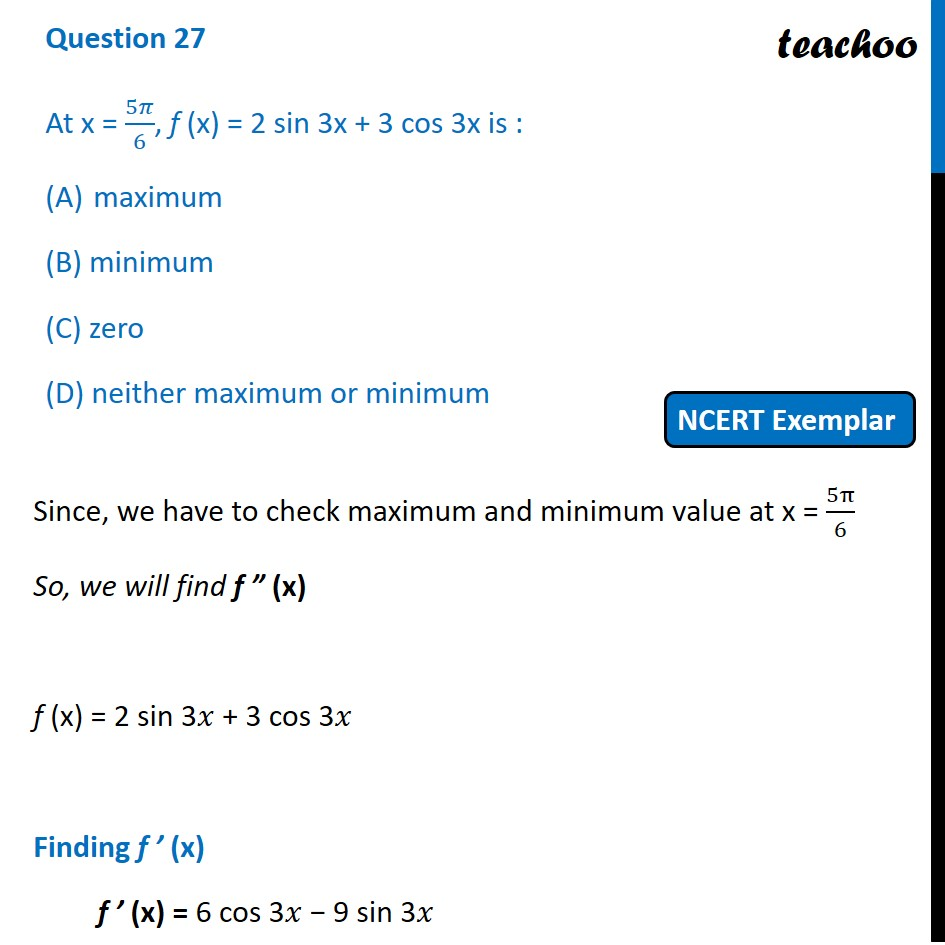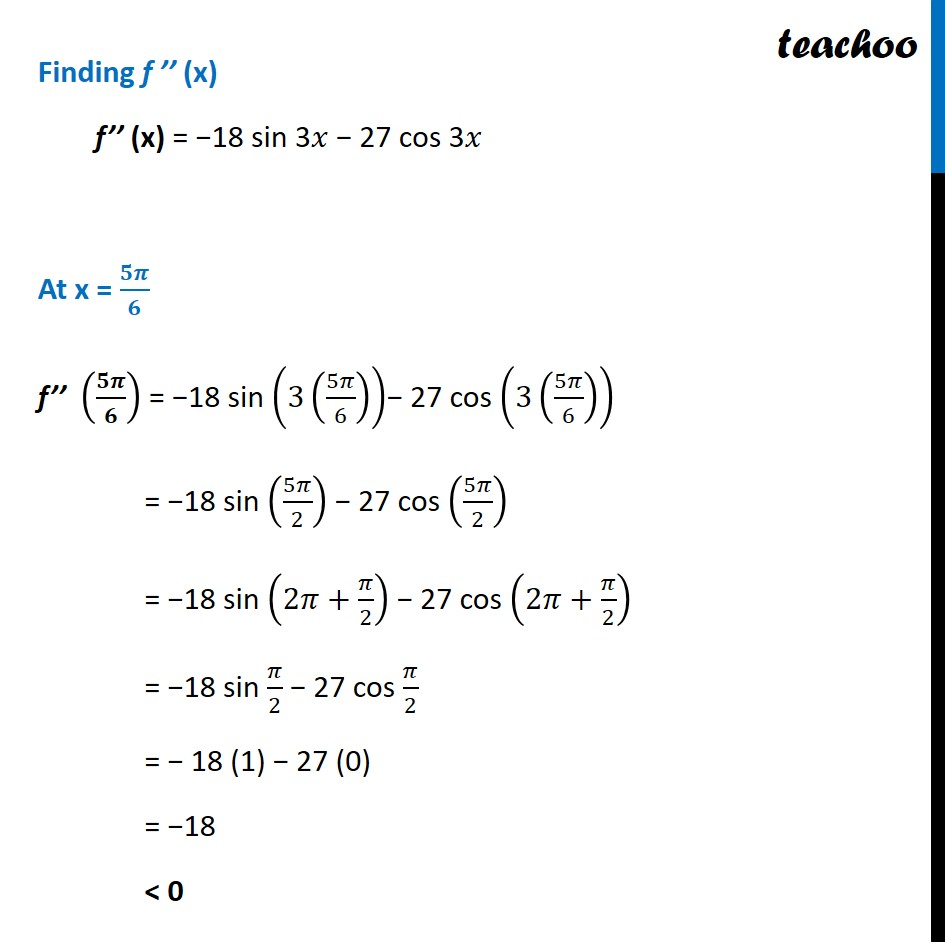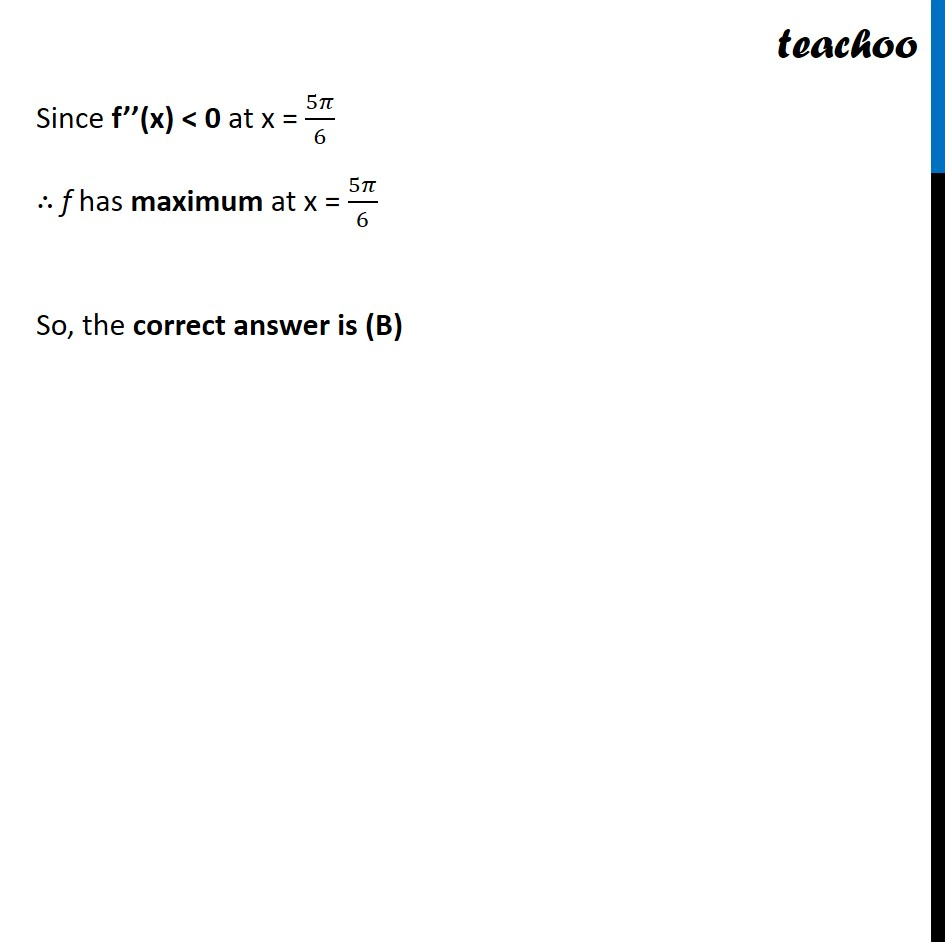NCERT Exemplar - MCQs

Chapter 6 Class 12 Application of Derivatives
Serial order wise

## (D) neither maximum or minimumLearn in your speed, with individual attention - Teachoo Maths 1-on-1 Class

### Transcript

Question 14 At x = 5𝜋/6, f (x) = 2 sin 3x + 3 cos 3x is : maximum (B) minimum (C) zero (D) neither maximum or minimum Since, we have to check maximum and minimum value at x = 5π/6 So, we will find f ” (x) f (x) = 2 sin 3𝑥 + 3 cos 3𝑥 Finding f ’ (x) f ’ (x) = 6 cos 3𝑥 − 9 sin 3𝑥 Finding f ’’ (x) f’’ (x) = −18 sin 3𝑥 − 27 cos 3𝑥 At x = 𝟓𝝅/𝟔 f’’ (𝟓𝝅/𝟔) = −18 sin (3(5𝜋/6))− 27 cos (3(5𝜋/6)) = −18 sin (5𝜋/2) − 27 cos (5𝜋/2) = −18 sin (2𝜋+𝜋/2) − 27 cos (2𝜋+𝜋/2) = −18 sin 𝜋/2 − 27 cos 𝜋/2 = − 18 (1) − 27 (0) = −18 < 0 Since f’’(x) < 0 at x = 5𝜋/6 ∴ f has maximum at x = 5𝜋/6 So, the correct answer is (B)